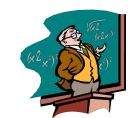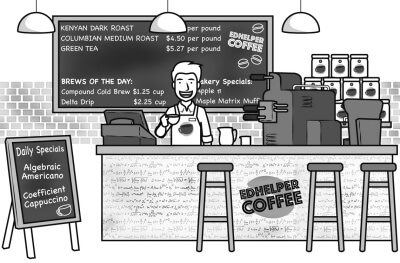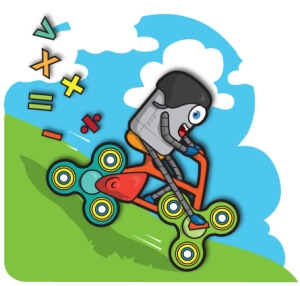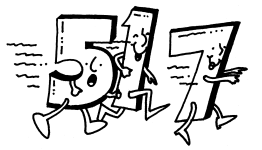Worksheets and No Prep Teaching Resources
Math Worksheets

# Middle School Math Worksheets

Middle school teachers will love the math worksheets that will excite, enrich, challenge, and instruct kids in your class. Use for fast finishers, homework assignments, or for something different in your classroom.Middle School Math Packet
Middle School Jumbo Math Worksheets Book
New Math Worksheets for Middle School Each Month

Middle School Math Mixed Review: Course 1
Level 1: Easiest (First 2 months of school)
Level 2: Next 3 months of school
Level 3: Next 3 months of school
Level 4: Entire course (Final months of school)

Middle School Math Mixed Review: Course 2-3
Level 1: Easiest (First 2 months of school)
Level 2: Next 3 months of school
Level 3: Next 3 months of school
Level 4: Entire course (Final months of school)

Algebra Math Challenge WorkbooksAlgebra Math Challenge

Algebra Math Challenge (Level 1 and 2 - More Difficult)

Middle School Math Challenge WorkbooksMiddle School Math Challenge Workbooks

Weekly Word Problems
Course 1
Last week's course 1 math word problems
This week's course 1 math word problems
Next week's course 1 math word problems

Course 2-3
Last week's course 2-3 math word problems
This week's course 2-3 math word problems
Next week's course 2-3 math word problems

Daily Review
Daily Review: Customize math review skills with word problems, language arts, and science

Weekly Word Problems in Spanish
Spanish - Middle School Math: Course 1
Spanish - Middle School Math Course 1: Last week's word problems
Spanish - Middle School Math Course 1: This week's word problems
Spanish - Middle School Math Course 1: Next week's word problems

Spanish - Middle School Math: Course 2 and Pre-Algebra
Spanish - Middle School Math Course 2 and Pre-Algebra: Last week's word problems
Spanish - Middle School Math Course 2 and Pre-Algebra: This week's word problems
Spanish - Middle School Math Course 2 and Pre-Algebra: Next week's word problems

edHelperKu Puzzles

Runaway Math Puzzles
 Runaway Math PuzzlesEquation Unknowns Puzzle

Build a Mixed Review Worksheet!

Select the skills to include:
 Place Value Whole Numbers Collect and Display Data Addition Subtraction Multiplication Division by 1-Digit Division by 2-Digits Order of Operations (positive whole numbers) Add and Subtract Decimals Multiply Decimals Divide Decimals Algebraic Reasoning Beginning Algebra Number Theory Fractions Measurement Integers Ratios and Proportional Reasoning Percents Repeating Decimals Plane Figures Lines and Angles Area and Perimeter Coordinate Graphing Perimeter, Circumference, and Area (easier) Perimeter, Circumference, and Area Volume and Surface Area Decimals Inequalities Probability

Number of Pages (for each key)
 Select the number of pages: 1 page 2 pages 3 pages 4 pages 5 pages 6 pages

Number of Keys
 Select the number of different printables: 1 key 2 keys 3 keys

Include an answer key (answer keys will be at the end of the printable)

Whole Numbers
Comparing whole numbers
Ordering whole numbers
Multiplication and division
Rounding whole numbers
Writing numbers with exponents
Comparing exponents
Order of operations
Distributive property
Patterns
Whole Numbers Review Printable

Whole Numbers - Word Problems
Biology
Geology
Physics
Chemistry
Sports
Whole Numbers Word Problems Mixed Review

Algebraic Reasoning
Order of Operations (3-4 numbers, no parenthesis)
Order of operations: simplify
Order of operations: fill in the missing number (1 missing)
Order of operations: fill in the missing operation (1 missing)
Order of operations: fill in all of the numbers
Order of operations: fill in all of the operations
Order of Operations (3-5 digits)
Order of operations: simplify
Order of operations: fill in the missing number (1 missing)
Order of operations: fill in the missing operation (1 missing)
Order of operations: fill in all of the numbers
Order of operations: fill in all of the operations
Algebraic Expressions
Evaluate expressions
More evaluate expressions
Writing algebraic expressions
Solving Equations
Solving subtraction equations
Solving multiplication and division equations
Solving addition, subtraction, multiplication, and division equations
Write an equation for each problem. Then solve the equation.
Functions
Complete the function table (rule and easier inputs given)
Complete the function table (rule and inputs given)
Complete the function table (missing some inputs and some outputs)
Write the rule for the function (all the inputs and outputs given)
Write the rule and fill in missing inputs or outputs
Algebraic Expressions Mixed Review
Algebraic Expressions Mixed Review

Beginning Algebra
Evaluate expressions
Writing algebraic expressions
Writing algebraic expressions (compound expressions)
Combining like terms
Solving subtraction equations
Solving addition and subtraction equations (including 3+ terms)
Solving multiplication and division equations
Solving equations (mix)
Beginning Algebra Word Problems Printable
Beginning Algebra Review Printable

Decimals
Comparing decimals
Comparing decimals (includes negatives)
Ordering decimals
Ordering decimals (includes negatives)
Write the number in scientific notation
Scientific notation - rewrite in decimal form
Solving simple addition and subtraction equations with decimals
Multiply decimals
Dividing decimals by whole numbers
Dividing decimals by decimals
Order of operations with decimals
Solving simple multiplication and division equations with decimals
Open-ended decimal questions
Decimals Word Problems Printable
Decimals Word Problems Printable: Science Problems (Physics, Biology, and Chemistry)
Decimals Word Problems Printable: Scientific Notation
Decimals Review Printable

Prime Numbers and Prime Factorization

Number Theory
Prime or composite
Divisibility: complete the table
Divisibility: fill in the missing digit
List all of the factors
Prime factorization
Greatest common factor
Least common multiple
Open-ended number theory questions
Number theory review printable

Number Properties
Number properties
Associative Property
Commutative Property
Distributive Property

Fractions
Equivalent fractions
Simplify fractions
Comparing fractions
Ordering fractions
Write improper fractions as mixed numbers
Write mixed numbers as improper fractions
Fill in the missing number
Multiplying fractions by whole numbers
Multiplying fractions
Multiplying mixed numbers
Dividing fractions
Dividing mixed numbers
Adding and subtracting unlike denominators (whole numbers)
Adding and subtracting unlike denominators (mixed numbers)
Solving addition and subtraction equations (algebra)
Solving multiplication and division equations (algebra)
Fractions Word Problems Printable
Fractions review printable

Fractions - Word Problems
General Fractions Word Problems
Biology
Geology
Physics
Chemistry
Sports
Fractions Word Problems Mixed Review

Repeating Decimals
Write a fraction as a decimal
Terminating decimals only (denominators of 2-20)
Repeating decimals only (denominators of 2-20)
Terminating and repeating decimals (denominators of 2-20)
Write a fraction (all in lowest terms) as a decimal (easier fractions)
Write a fraction as a decimal (larger denominators)
Write a fraction (all in lowest terms) as a decimal
Write a fraction as a decimal
Write a fraction as a decimal (all in lowest terms)
Write a decimal as a fraction
Write terminating decimals as fractions
Write repeating decimals as fractions (includes instructions)
Write repeating decimals as fractions
Ordering
Order 3 numbers
Order 7 numbers
Order 7 numbers (includes negatives)
Repeating Decimals Mixed Review

Measurement
Customary units of measure
Customary units of measure (with decimals)
Customary units of measure (with fractions)
Metric units of length
Metric units of mass
Metric units of capacity
Metric units of capacity (with metric tons)
Metric units of capacity (mixed - no metric tons)
Metric units of capacity (mixed)
Unit Conversions (chart)
Unit Conversions (convert quantity)
Adding and subtracting customary units of measure
Adding and subtracting customary units of measure (with fractions)
Ordering Metric units
Measurement review printable

Integers Unit
Representing integers
Ordering integers
Addition and subtraction (only 2 numbers each)
Multiplication and Division (only 2 numbers each)
Addition, subtraction, multiplication, and division mix
Fill in the missing negative signs
Finding absolute value
Comparing integers
Solving multiplication and division (algebra)
Solving equations (algebra)
Addition and subtraction with decimals (only 2 numbers each)
Multiplication and Division with decimals (only 2 numbers each)
Mix with decimals
Fill in the missing negative signs
Open-ended integer problems
Word Problems
Word Problems (science)
Word Problems (sports)
Integers Review Printable

Inequalities
Determine the truth of an inequality
Solving inequalities by adding or subtracting (whole numbers)
Solving inequalities by adding or subtracting (fractions)
Solving Inequalities by multiplying or dividing
Solving Multi-Step Inequalities
Inequalities Word Problems Printable
Inequalities Review Printable

Ratios and Proportions
State whether the ratios are proportional
Solving proportions (no decimals)
Solving proportions (decimals)
Create a proportion from set of numbers
Unit Conversions (chart)
Unit Conversions (convert quantity)
Unit Rates
Scale Factors
Similar Figures
Ratios and Proportions Word Problems Printable
Ratios and Proportions Review Printable

Ratios - Word Problems
General Ratios Word Problems
Biology
Geology
Physics
Chemistry
Sports
Ratios Word Problems Mixed Review

Percents Unit
Write percent as fraction
Write percent as decimal
Write decimal as percent
Write fraction as percent
Write fraction as percent (including irrational fractions)
Fill in missing value (2 items)
Fraction, decimal, and percent table
Percent of a number
Solve for percent of a number
Solve for percent change
Simple Interest (whole years and whole interest rates only)
Simple Interest
Percents Word Problems Printable
Percents Review Printable

Percents - Word Problems
General Percents Word Problems
Biology
Geology
Physics
Chemistry
Sports
Percents Word Problems Mixed Review

Perimeter

Area

Perimeter, Circumference, and Area
Find area of triangles (given coordinates)
Find area of rectangles and squares (given coordinates)
Find area of trapezoids (given coordinates)
Find area review (given coordinates)
Area and perimeter word problems: rectangles and parallelograms
Area and perimeter: rectangles with fractions
Area and perimeter of rectangles (algebra)
Area and perimeter: triangles
Area and perimeter: trapezoids
Area and perimeter: triangles (with fractions)
Area and perimeter: trapezoids (with fractions)
Pythagorean theorem
Circles: Find the circumference
Circles: Find the area
Circles: Find the circumference and area
Perimeter and circumference - Word Problems Printable
Area - Word Problems Printable
Perimeter, Circumference, and Area Review Printable

Volume and Surface Area
Surface area and volume of right rectangular prisms
Volume of prisms and cylinders (Graphics)
Volume of cones, pyramids, and spheres (Graphics)
Volume of prisms, cylinders, cones, pyramids, and spheres
Surface area of prisms and cylinders
Surface area of pyramids, cones, and spheres
Volume Word Problems Printable
Volume and Surface Area Review Printable

Probability
Counting principle
Theoretical probability (write answer as a fraction)
Theoretical probability (write answer as a decimal)
Theoretical probability (write answer as a percent)
Theoretical probability
Compound events (independent events)
Compound events (dependent events)
Compound events
Combinations
Permutations
Combinations and permutations
Word problems - experimental probability
Word problems - theoretical probability
Word problems - compound events
Word problems - combinations and permutations
Word problems review
Probability Review Printable

Statistics - Word Problems
Biology
Geology
Physics
Chemistry

Algebra
Visit the edHelper.com algebra section for algebra materials

Algebra Mixed Review Worksheet
For edHelper.com grades 9-12 subscribers. -

Select the skills to include:
 Equations Exponents Linear Equations Systems of Linear Equations Inequalities Functions Matrices Simplify Algebraic Fractions Polynomials Factoring Polynomial Functions Radical Numbers Advanced Radical Numbers Quadratic Equations Conics Word Problems

 Select the number of pages: 1 page 2 pages 3 pages 4 pages 5 pages 6 pages

Teacher Recommendations:  Worksheet and Lesson Plan Activity Ideas

Math

Poetry

Money

Handwriting

Science

Graphic Organizers

Weather

Time

Plants

Main Idea

Calendar

Spelling

Shapes

More Activities, Lesson Plans, and Worksheets

Have a suggestion or would like to leave feedback?